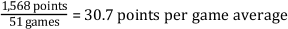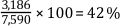# Statistics

﻿

## LabVIEW 2018ヘルプ

### ダウンロード (Windowsのみ)LabVIEW 2016ヘルプLabVIEW 2017ヘルプLabVIEW 2018ヘルプLabVIEW 2019ヘルプLabVIEW 2020ヘルプ

Statistics allow you to summarize data and draw conclusions for the present by condensing large amounts of data into a form that brings out all the essential information and is yet easy to remember. To condense data, single numbers must make the data more intelligible and help draw useful inferences. For example, in a season, a sports player participates in 51 games and scores a total of 1,568 points. The total of 1,568 points includes 45 points in Game A, 36 points in Game B, 51 points in Game C, 45 points in Game D, and 40 points in Game E. As the number of games increases, remembering how many points the player scored in each individual game becomes increasingly difficult. If you divide the total number of points that the player scored by the number of games played, you obtain a single number that tells you the average number of points the player scored per game. The following equation yields the points per game average for the player.Computing percentage provides a method for making comparisons. For example, the officials of an American city are considering installing a traffic signal at a major intersection. The purpose of the traffic signal is to protect motorists turning left from oncoming traffic. However, the city has only enough money to fund one traffic signal but has three intersections that potentially need the signal. Traffic engineers study each of the three intersections for a week. The engineers record the total number of cars using the intersection, the number of cars traveling straight through the intersection, the number of cars making left-hand turns, and the number of cars making right-hand turns. The following table shows the data for one of the intersections.

Day Total Number of Cars Using the Intersection Number of Cars Turning Left Number of Cars Turning Right Number of Cars Continuing Straight
1 1,258 528 330 400
2 1,306 549 340 417
3 1,355 569 352 434
4 1,227 515 319 393
5 1,334 560 346 428
6 694 291 180 223
7 416 174 108 134
Totals 7,590 3,186 1,975 2,429

Looking only at the raw data from each intersection might make determining which intersection needs the traffic signal difficult because the raw numbers can vary widely. However, computing the percentage of cars turning at each intersection provides a common basis for comparison. To obtain the percentage of cars turning left, divide the number of cars turning left by the total number of cars using the intersection and multiply that result by 100. For the intersection whose data is shown in the previous table, the following equation gives the percentage of cars turning left.Given the data for the other two intersections, the city officials can obtain the percentage of cars turning left at those two intersections. Converting the raw data to a percentage condenses the information for the three intersections into single numbers representing the percentage of cars that turn left at each intersection. The city officials can compare the percentage of cars turning left at each intersection and rank the intersections in order of highest percentage of cars turning left to the lowest percentage of cars turning left. Ranking the intersections can help determine where the traffic signal is needed most. Thus, in a broad sense, the term statistics implies different ways to summarize data to derive useful and important information from it.

﻿

﻿